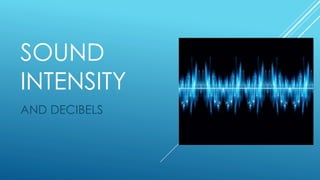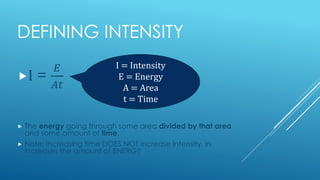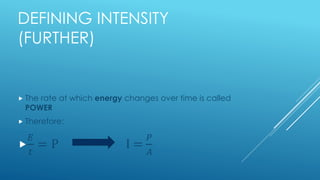Successfully reported this slideshow.

# Sound Intensity

6

Share×
1 of 12
1 of 12

# Sound Intensity

6

Share

Sound intensity

#### Transcript

1. 1. SOUND INTENSITY AND DECIBELS
2. 2. DEFINING INTENSITY I = 𝐸 𝐴𝑡  The energy going through some area divided by that area and some amount of time.  Note: increasing time DOES NOT increase intensity, in increases the amount of ENERGY I = Intensity E = Energy A = Area t = Time
3. 3. DEFINING INTENSITY (FURTHER)  The rate at which energy changes over time is called POWER  Therefore:  𝐸 𝑡 = P I = 𝑃 𝐴
4. 4. DEFINING INTENSITY (FURTHERER)  Units of Power = Watts /Units of Area = meters squared  Therefore: intensity is in units of W/m2
5. 5. FORMULA FOR INTENSITY  Sound travels in a SPHERE, and not in a circle.  Formula for the surface area of a sphere is:  4𝜋𝑟2  Therefore: I = 𝑃 4𝜋𝑟2 Surface area of a sphere with radius r
6. 6. RANGE OF HEARING (IN GENERAL)  Pain threshold of hearing: 1 W/m2  Lowest sound humans can hear: 1 x 10-12 W/m2  These sounds are vibrating at less than a width of one molecule! Wow!  Reference intensity  Io  RANGE OF HEARING: 1013Io
7. 7. DECIBELS  The range of 1013Io (10,000,000,000,000) is far too large to be used efficiently  We use DECIBELS as a more efficient range  Decibels (dB) are units for logarithmic comparisons of intensity levels(loudness)
8. 8. INTENSITY LEVEL EQUATION  We want to compare a certain intensity (I) to the faintest sound a human can possibly hear (Io)  𝐼 𝐼 𝑜  this is a dimensionless number  This number is in between the previous given range of (10,000,000,000,000)  Since the decibel range is a logarithmic scale, we use log10 on the given number.
9. 9. INTENSITY LEVEL EQUATION (MORE)  So far: dB = log10( 𝐼 𝐼 𝑜 )  Because they have been dubbed decibels, the equation needs to multiplied by 10 (dec- stems from the Greek root, meaning ten)  Now: dB = 10log10[ 𝐈 𝐈 𝐨 ]
10. 10. SOLVING FOR INTENSITY (I)  dB = 10log10[ I Io ]  Need to get rid of the 10 on the right hand term, so:  𝑑𝐵 10 = log10[ I Io ]  To get rid of the log10 on the right hand side, raise both sides to the tenth power:  10dB/10 = [ I Io ]  To isolate for I, multiply both sides by Io:  Io10dB/10 = I
11. 11. THANK YOU FOR READING! AARON REYES PHYS 101 202
12. 12. WORKS CITED  http://hyperphysics.phy-astr.gsu.edu/hbase/sound/intens.html  Physics for Scientists and Engineers: an Interactive Approach: Revised Custom Volume 1 – PHYS 101. Hawkes, Iqbal, et al. Toronto, Ontario. 2015. Page 251

Sound intensity

#### Transcript

1. 1. SOUND INTENSITY AND DECIBELS
2. 2. DEFINING INTENSITY I = 𝐸 𝐴𝑡  The energy going through some area divided by that area and some amount of time.  Note: increasing time DOES NOT increase intensity, in increases the amount of ENERGY I = Intensity E = Energy A = Area t = Time
3. 3. DEFINING INTENSITY (FURTHER)  The rate at which energy changes over time is called POWER  Therefore:  𝐸 𝑡 = P I = 𝑃 𝐴
4. 4. DEFINING INTENSITY (FURTHERER)  Units of Power = Watts /Units of Area = meters squared  Therefore: intensity is in units of W/m2
5. 5. FORMULA FOR INTENSITY  Sound travels in a SPHERE, and not in a circle.  Formula for the surface area of a sphere is:  4𝜋𝑟2  Therefore: I = 𝑃 4𝜋𝑟2 Surface area of a sphere with radius r
6. 6. RANGE OF HEARING (IN GENERAL)  Pain threshold of hearing: 1 W/m2  Lowest sound humans can hear: 1 x 10-12 W/m2  These sounds are vibrating at less than a width of one molecule! Wow!  Reference intensity  Io  RANGE OF HEARING: 1013Io
7. 7. DECIBELS  The range of 1013Io (10,000,000,000,000) is far too large to be used efficiently  We use DECIBELS as a more efficient range  Decibels (dB) are units for logarithmic comparisons of intensity levels(loudness)
8. 8. INTENSITY LEVEL EQUATION  We want to compare a certain intensity (I) to the faintest sound a human can possibly hear (Io)  𝐼 𝐼 𝑜  this is a dimensionless number  This number is in between the previous given range of (10,000,000,000,000)  Since the decibel range is a logarithmic scale, we use log10 on the given number.
9. 9. INTENSITY LEVEL EQUATION (MORE)  So far: dB = log10( 𝐼 𝐼 𝑜 )  Because they have been dubbed decibels, the equation needs to multiplied by 10 (dec- stems from the Greek root, meaning ten)  Now: dB = 10log10[ 𝐈 𝐈 𝐨 ]
10. 10. SOLVING FOR INTENSITY (I)  dB = 10log10[ I Io ]  Need to get rid of the 10 on the right hand term, so:  𝑑𝐵 10 = log10[ I Io ]  To get rid of the log10 on the right hand side, raise both sides to the tenth power:  10dB/10 = [ I Io ]  To isolate for I, multiply both sides by Io:  Io10dB/10 = I
11. 11. THANK YOU FOR READING! AARON REYES PHYS 101 202
12. 12. WORKS CITED  http://hyperphysics.phy-astr.gsu.edu/hbase/sound/intens.html  Physics for Scientists and Engineers: an Interactive Approach: Revised Custom Volume 1 – PHYS 101. Hawkes, Iqbal, et al. Toronto, Ontario. 2015. Page 251

## More Related Content

### Related Books

Free with a 30 day trial from Scribd

See all

### Related Audiobooks

Free with a 30 day trial from Scribd

See all